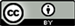1. 引言

2. 坐标系的建立2.1. 机体坐标系

{ x i b = d i cos ( φ i − π / 4 ) y i b = d i sin ( φ i − π / 4 ) (1)

x b , y b 是机体坐标系下x, y的坐标值。

2.2. 大地坐标系

{ x i w = x i b cos θ − y i b sin θ y i w = x i b sin θ + y i b cos θ (2)

x w , y w 表示大地坐标系下的x, y的坐标值(图2)。

3. 环境中特征线段的提取

P = { S 1 , S 2 , S 3 , ⋯ , S M }

(1) 根据实际需要，确定一个点和线段关系的阈值。

(2) 点集 S i 作为待处理数据点，连接待处理数据点的起始点和终止点，建立一个线段的模型。

(3) 找到待处理数据点中其它数据点距离线段的距离，记录其最大值，判断这个最大值与阈值的关系，如果最大值小于阈值，则不进行分割，否则，把线段分为两部分。

(4) 判断点集 S i 中的线段是否处理完，如果没有，跳到第(2)步。

4. 改进的EKF算法4.1. 特征表示与选择

4.2. 模型的建立

4.2.1. 观测模型的建立

h i ( X , M ) = ( r i − x 2 + y 2 cos ( β − α i ) α i − θ ) (3)

h i ( X , M ) = ( r i − x 2 + y 2 cos ( β − α i ) ) (4)

H i = [ H 11 H 12 ] (5)

H 11 = − x ρ cos ( β − α i ) − y ρ sin ( β − α i )

H 12 = − y ρ cos ( β − α i ) + x ρ sin ( β − α i )

ρ = x 2 + y 2

{ K k , i = P k | k , i − 1 H i T ( H i P k | k , i − 1 H i T + R i ) x k | k − 1 , i = x k | k − 1 , i + K k , i ( Y i − h i ( X , M ) ) P k | k , i = ( I − K k , i H i ) P k | k , i − 1 (6)

4.2.2. 过程模型的建立

{ x k | k − 1 = x k − 1 | k − 1 + cos θ k Δ x − sin θ k Δ y y k | k − 1 = y k − 1 | k − 1 + sin θ k Δ x + cos θ k Δ y (7)

5. 实验结果分析

6. 结论